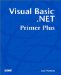# Shorthand Operators

Certain types of arithmetic operations are performed a lot in most computer programs. These common arithmetic operations perform some arithmetic operation on the original value of a variable and then reassign the new value back to the original variable. A common example is the statement that increments a variable by 1, which is written as follows :

` Num = 10  ' Do some other stuff... Num = Num + 1    ' This increments Num by 1 `

In the last statement, you take the original value of Num (that is, 10 ), add 1 to it, and then assign the new value back to Num . In short, the last statement simply increments Num by 1.

The increment statement, Num = Num + 1 , has this general form:

`  Operand1  =  Operand1 ArithmeticOperator Operand2  `

Because such operations are so common, Visual Basic .NET supplies shorthand operators to simplify these statements. With a shorthand operator, the increment statement can be rewritten as this:

` Num += 1 `

The effect on Num is identical; its value is incremented by 1.

As you can see, the shorthand operator replaces this syntax:

`  Operand1  =  Operand1 ArithmeticOperator Operand2  `

with this:

`  Operand1 ArithmeticOperator  =  Operand2  `

The only catch is that the arithmetic shorthand operators must do the assignment into themselves . Whereas Operand2 can be a variable, a numeric constant, or another expression, Operand1 must be a variable. The shorthand operators are listed in Table 9.2.

##### Table 9.2. Shorthand Arithmetic Operators

Shorthand Operator

Operation

Full Expression

Shorthand Expression

`  +=  `

X = X + 1

X += 1

`  -=  `

Subtraction

X = X + Y

X -= Y

`  *=  `

Multiplication

X = X * 8

X *= 8

`  /=  `

Division

X = X / 2

X /= 2

`  \=  `

Integer Division

X = X \ 3

X \= 3

`  ^=  `

Exponentiation

X = X ^ 2

X ^= 2

The shorthand operators don't give you anything you didn't have before. They simply give you a shorter way to write the statements. (By the way, don't forget the shorthand operator for string concatenation, &= , for building strings.)Visual Basic .NET Primer Plus
ISBN: 0672324857
EAN: 2147483647
Year: 2003
Pages: 238
Authors: Jack Purdum

Similar book on Amazon